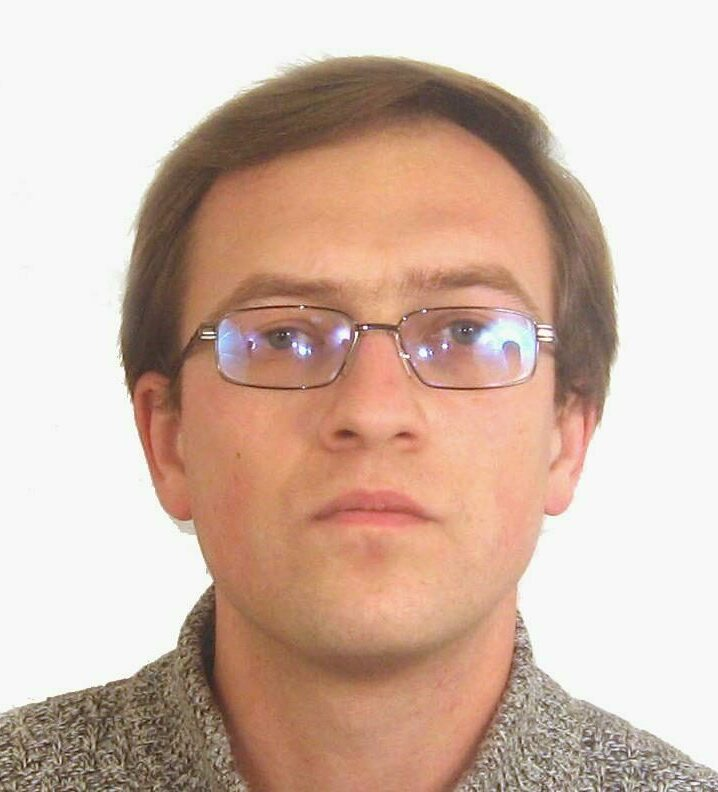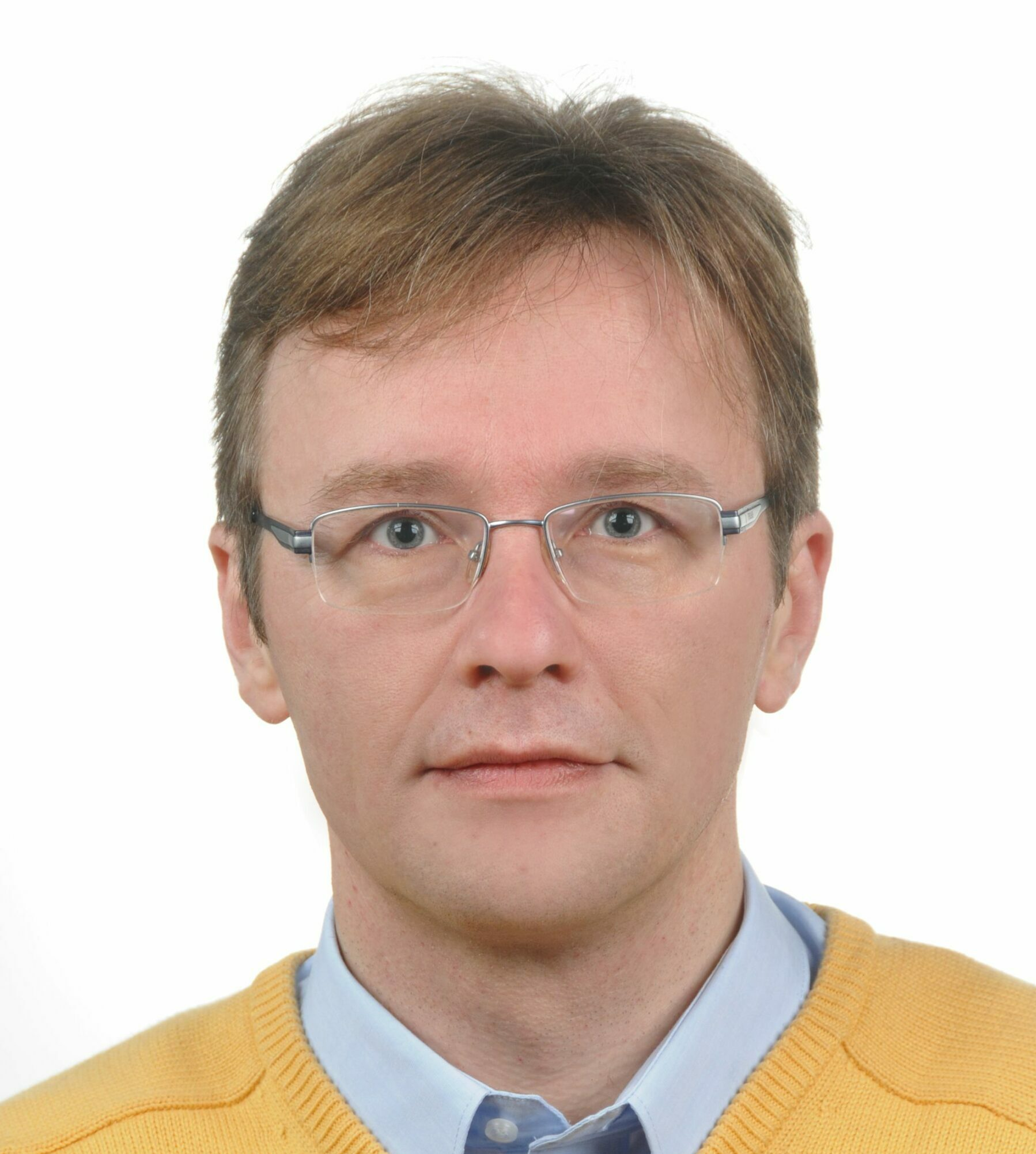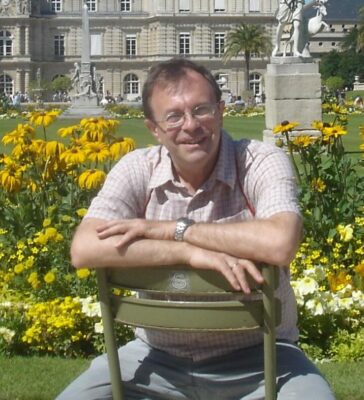# School "Complex analysis and Operator Theory"

29 November 2021 to 3 December 2021
Online
Europe/Moscow timezone

# ‎Complex Analysis, Combinatorics and Operator Theory

### November 29 – December 3, 2021

An advanced school for young researchers featuring three minicourses in vibrant areas of mathematics.  The target audience includes graduate, master and senior bachelor students of any mathematical specialty.

## Lecturers:Ilya Shkredov Institute of mathematics, RASYuriy Tomilov Institute of mathematics, PANAlexander Volberg Michigan State University

## Courses:

### Additive combinatorics and analysis (Ilya Shkredov)

Additive combinatorics is an actively developing area of math, that lies at the intersection of Number theory and Combinatorics. It also involves extensive use of techniques from Analysis, Graph theory, Ergodic theory, Probability, Algebraic geometry, Topology and Geometry of numbers.

In this course we discuss classic applications (Lectures 1, 2, 4) of functional-theoretic methods to additive combinatorics (setting aside arithmetic combinatorics and non-commutative approaches), and, vice versa, application of additive combinatorics to some questions from function theory (Lecture 3).

Lecture 1: Additive combinatorics: discrete Fourier transform, Roth theorem, sets without solutions of linear equations

Lecture 2: Additive combinatorics: Gowers uniformity norms, corners problem, transference principle (for primes and Sidon sets)

Lecture 3: Additive combinatorics: integer sets with small Wiener norm on integers, Littlewood problem, additive energies, additive dimension and dissociativity

Lecture 4: Additive combinatorics: almost periodicity of convolutions, Croot-Sisask theorem, applications to linear equations and sets with small doubling

### Bounded functional calculi for unbounded operators (Yuriy Tomilov)

Functional calculi is a classical subject of operator theory, unifying such distant areas as complex analysis, harmonic analysis and PDE. Several breakthroughs in analysis, e.g.  solving the famous Kato square root problem or obtaining the L^2-boundedness of Cauchy integrals on Lipschitz curves, depend on the functional calculi theory. Moreover, the theory became indispensable nowadays for a number of applied problems, stemming mostly from evolution equations.

Roughly, the functional calculi theory provides  a way to assign a linear operator A on a Banach space a coherent family operators f(A), when f runs through a function algebra. So it can be considered as an operator version of function theory. The major issue is how to provide a good control'' of f(A) given A and f,  and we just touch this problem putting aside a number of modern developments around it.

We'll start the course with a short overview of the theory of  functional calculi, putting an emphasis on generators of strongly continuous operator semigroups. This class of operators is of major importance for the study of partial differential equations, and it will be the main object of our studies as well. Then we'll proceed with introducing the so-called B-calculus for generators of Hilbert space semigroups and generators of holomorphic Banach space semigroups developed  recently in collaboration with C. Batty (Oxford) and A. Gomilko (Torun). We'll explain its background, underline its attractive features and  provide several applications. Being a far-reaching generalisation of the classical Hille-Phillips functional calculus, the B-calculus appeared to be very handy for norm-estimates of various operator functions. This will be one of the main points of the course.
If time permits, we'll discuss a very recent generalisation of the B-calculus due to L. Arnold and  C. Le Merdy, and mention a couple of related open problems.

Lecture 1: A panorama of functional calculi from the bird's eye view.

Lecture 2: Basics of operator semigroups, their generators and related matters.

Lecture 3: Introduction to the B-calculus: motivation, properties and related function theory.

Lecture 4: Some applications of the B-calculus and its further perspectives.

### Buffon needle (Alexander Volberg)

The probability of Buffon needle to land near Cantor set. Quantitative Besicovitch theorem.

One of classical theorems of Besicovitch claims that all self-similar Cantor sets of dimension 1 on the plane are  irregular in the sense of Besicovitch.  In particular, this means that the probability of Buffon needle to intersect such a set (conditioned to event that it intersected a disc contains this Cantor set) is zero. This immediately  implies that  the probability of Buffon needle to intersect the $\delta$-neighborhood of such Cantor set tends to zero when $\delta$ tends to zero. But how fast?

The attempts to clarify this (undertaken with Michael Bateman, Matt Bond, Izabella Łaba, Fedor Nazarov and Yuval Peres in various combinations) reveal the unexpected relations of this question to Fourier and Complex analysis, Combinatorics (including a bit of additive combinatorics), Algebra, Diophantine equations and Number theory in the form of Gelfond—Baker theory initiated by Hilbert’s 7th problem.

I hope to show this plethora of methods that we aimed to proving what we called a power estimate’’, namely that the above probability is at most $|\log\delta|^{-p}$  with some positive $p$. However, we managed to get such power estimate only if the base of Cantor set is 3 and 4, and, if the base is at least 5,  for some Cantor sets with very special algebraic properties. It is still much better than previous estimates of Peres—Solomyak. The problem turned out to be related to such combinatorial questions as: count the number of intersections of diagonals inside a regular polygon (Poonen—Rubinstein), and such algebraic questions as: describe families of roots of unity, whose sum vanishes (Rédei, de Brujin, Schoenberg, Mann, Lam, Leung).

Lecture 1: Estimate from below for the Buffon needle probability of the four-corner Cantor set

Lecture 2: Fourier analysis for the estimate from above for the Buffon needle probability of the Cantor-type set

Lecture 3: Complex analysis, Riesz products for the Buffon needle's quasi singular directions

Lecture 4: Combinatorics: why the measure of quasi singularity directions is rather small

Lecture 5: Algebra and Number theory: cyclotomic polynomials and Linear Multi-Polygon Relations, Gelfond-Baker theorem and Hilbert's 7th problem

## Institutions participating in the organization of the event:

Starts
Ends
Europe/Moscow
Online
https://us02web.zoom.us/j/4437261792
Registration
Registration for this event is currently open.